# Simple Pendulum

An ideal simple pendulum consists of a heavy point mass body suspended by a weightless, inextensible and perfectly flexible string from a rigid support about which it is free to oscillate.

But in reality neither point mass nor weightless string exist, so we can never construct a simple pendulum strictly according to the definition.

Let mass of the bob = m

Length of simple pendulum = l

Displacement of mass from mean position (OP) = x

When the bob is displaced to position P, through a small angle q from the vertical. Restoring force acting on the bobImportant Points

(i) The period of simple pendulum is independent of amplitude as long as its motion is simple harmonic. But if q is not small, sin q  ¹ q then motion will not remain simple harmonic but will become oscillatory. In this situation if q0 is the amplitude of motion. Time period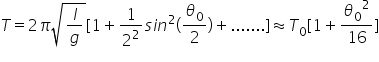(ii) Time period of simple pendulum is also independent of mass of the bob. This is why

(a) If the solid bob is replaced by a hollow sphere of same radius but different mass, time period remains unchanged.

(b) If a girl is swinging in a swing and another sits with her, the time period remains unchanged.

(iii) Time period  where l is the distance between point of suspension and center of mass of bob and is called effective length.

(a) When a sitting girl on a swinging swing stands up, her center of mass will go up and so l and hence T will decrease.

(b) If a hole is made at the bottom of a hollow sphere full of water and water comes out slowly through the hole and time period is recorded till the sphere is empty, initially and finally the center of mass will be at the center of the sphere. However, as water drains off the sphere, the center of mass of the system will first move down and then will come up. Due to this l and hence T first increase, reaches a maximum and then decreases till it becomes equal to its initial value.

(iv) If the length of the pendulum is comparable to the radius of earth then(v) If the bob of simple pendulum is suspended by a wire then effective length of pendulum will increase with the rise of temperature due to which the time period will increase.(vi) If bob a simple pendulum of density r is made to oscillate in some fluid of density s (where s <r) then time period of simple pendulum gets increased.

As thrust will oppose its weight therefore(vii) If a bob of mass m carries a positive charge q and pendulum is placed in a uniform electric field of strength E directed vertically upwards.

In given condition net down ward accelerationIf the direction of field is vertically downward then time period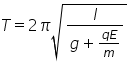(viii) Pendulum in a lift :  If the pendulum is suspended from the ceiling of the lift.

(a) If the lift is at rest or moving down ward /up ward with constant velocity.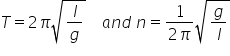(b) If the lift is moving up ward with constant acceleration a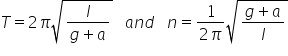Time period decreases and frequency increases

(c) If the lift is moving down ward with constant acceleration a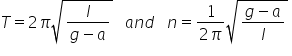\

Time period increase and frequency decreases

(d) If the lift is moving down ward with accelerationIt means there will be no oscillation in a pendulum.

Similar is the case in a satellite and at the centre of earth where effective acceleration becomes zero and pendulum will stop.

(ix) The time period of simple pendulum whose point of suspension moving horizontally with acceleration a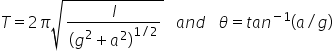(x) If simple pendulum suspended in a car that is moving with constant speed v around a circle of radius r.Putting  T = 2 sec  and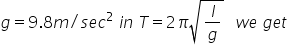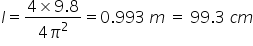Hence length of second’s pendulum is 99.3 cm or nearly 1 meter on earth surface.

For the moon the length of the second’s pendulum will be 1/6 meter [As ]

(xii) In the absence of resistive force the work done by a simple pendulum in one complete oscillation is zero.

(xiii) Work done in giving an angular displacement q  to the pendulum from its mean position.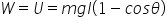(xiv) Kinetic energy of the bob at mean position = work done or potential energy at extreme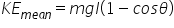(xv) Various graph for simple pendulum

Related Keywords